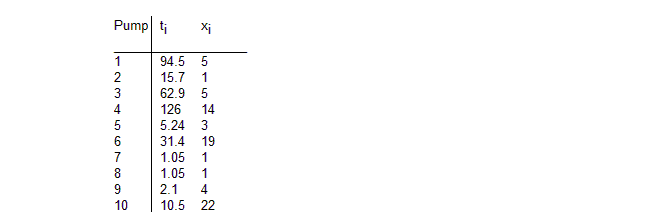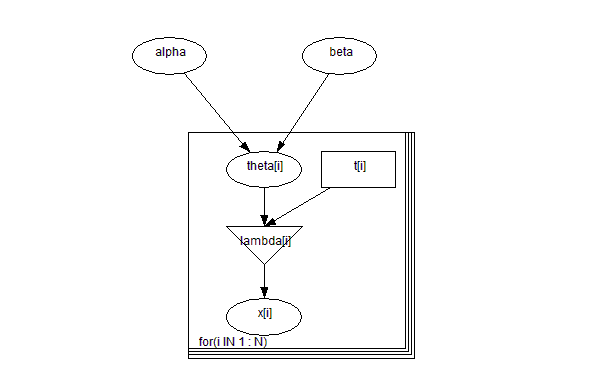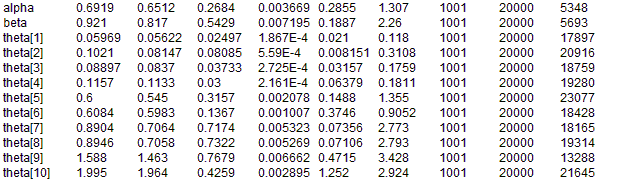#### Pumps: conjugate gamma-Poisson hierarchical model

George et al (1993) discuss Bayesian analysis of hierarchical models where the conjugate prior is adopted at the first level, but for any given prior distribution of the hyperparameters, the joint posterior is not of closed form. The example they consider relates to 10 power plant pumps. The number of failures xi is assumed to follow a Poisson distribution

xi ~ Poisson(θiti)   i = 1,...,10

where θi is the failure rate for pump i and ti is the length of operation time of the pump (in 1000s of hours). The data are shown below.A conjugate gamma prior distribution is adopted for the failure rates:

θi ~ Gamma(α, β), i = 1,...,10

George et al (1993) assume the following prior specification for the hyperparameters α and β

α ~ Exponential(1.0)
β ~ Gamma(0.1, 1.0)

They show that this gives a posterior for β which is a gamma distribution, but leads to a non-standard posterior for α. Consequently, they use the Gibbs sampler to simulate the required posterior densities.
Graphical model for pump example:BUGS language for pump example:

model
{
for (i in 1 : N) {
theta[i] ~ dgamma(alpha, beta)
lambda[i] <- theta[i] * t[i]
x[i] ~ dpois(lambda[i])
}
alpha ~ dexp(1)
beta ~ dgamma(0.1, 1.0)
}
##### Data
``` list(t = c(94.3, 15.7, 62.9, 126, 5.24, 31.4, 1.05, 1.05, 2.1, 10.5),   x = c( 5, 1, 5, 14, 3, 19, 1, 1, 4, 22), N = 10) ```
##### Inits for chain 1
``` list(alpha = 1, beta = 1) ```

##### Inits for chain 2
``` list(alpha = 10, beta = 10) ```
Results